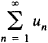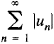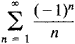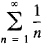Conditional Convergence

Also found in: Dictionary, Wikipedia.
Related to Conditional Convergence: absolute convergence

conditional convergence

[kən′dish·ən·əl kən′vər·jəns]
(mathematics)
The property of a series that is convergent but not absolutely convergent.
McGraw-Hill Dictionary of Scientific & Technical Terms, 6E, Copyright © 2003 by The McGraw-Hill Companies, Inc.
The following article is from The Great Soviet Encyclopedia (1979). It might be outdated or ideologically biased.

Conditional Convergence

a concept of mathematical analysis. The seriesis said to be conditionally convergent if it is convergent and the series(whose terms are the absolute values of the terms of the original series) is divergent.

For example, the seriesis conditionally convergent, since the absolute values of its terms form the divergent, harmonic seriesIf a series is conditionally convergent, then the two series consisting of its positive and negative terms, respectively, are divergent. According to Riemann’s theorem, by an appropriate rearrangement of the terms of a given conditionally convergent series we can obtain a divergent series or a series that has a prescribed sum. If two conditionally convergent series are multiplied term by term, a divergent series may result.

The concept of conditional convergence can be extended to series of vectors, to infinite products, and to improper integrals.

Mentioned in ?
References in periodicals archive ?
Keywords: Absolute convergence, Conditional convergence, Solow swan growth model, Pooled ordinary least square
The regression results confirm conditional convergence as the coefficient of the regression slope is negative, [[alpha].sub.1] =-0,032 (see Figure 4).
The fact that the creation of new knowledge is based on the existing stock of knowledge dates back to Romer (1990); [[beta].sub.0] may be interpreted as a factor of conditional convergence and expresses the catch-up effect towards the steady state.
(15.) Galor (2010) contends that club convergence is more plausible than a conditional convergence hypothesis if economies that are identical in their fundamentals converge to the same steady-state level of output per capita regardless of their initial conditions.
Conditional convergence (H2): House price convergence is associated with regional structures.
conditional convergence. (21) Because convergence is conditional on the
Many authors deal with the subject of convergence and usually two types of convergence between different economies can be defined; these are absolute convergence and conditional convergence. Absolute convergence implies that countries or regions with lower initial values of the capital/labor share show a higher growth rate of output per capita and tend to catch up with and converge to the countries or regions with a higher capital/labor share.
We also tested for conditional convergence. We use the share of services sector (ser) in total GDP (following the idea of Tsionas and Christopoulos, ) as the variable that serves as proxy for the steady state.
By using different pre-assumptions, it is possible to catalogue the model into three types, namely the regional absolute convergence model, the regional conditional convergence model and the regional club convergence model.
Therefore, they developed and tested a model of conditional convergence based on the specifications of the Solow model.
However, the data did support conditional convergence, which implies that different regions followed independent growth paths.

Site: Follow: Share:
Open / Close